# Menu driven program for all operations on singly linked list in C

• Difficulty Level : Easy
• Last Updated : 02 Feb, 2022

A Linked List is a linear data structure that consists of two parts: one is the data part and the other is the address part. In this article, all the common operations of a singly linked list is discussed in one menu-driven program.

Operations to be performed:

• createList(): To create the list with ‘n’ number of nodes initially as defined by the user.
• traverse(): To see the contents of the linked list, it is necessary to traverse the given linked list. The given traverse() function traverses and prints the content of the linked list.
• insertAtFront(): This function simply inserts an element at the front/beginning of the linked list.
• insertAtEnd(): This function inserts an element at the end of the linked list.
• insertAtPosition(): This function inserts an element at a specified position in the linked list.
• deleteFirst(): This function simply deletes an element from the front/beginning of the linked list.
• deleteEnd(): This function simply deletes an element from the end of the linked list.
• deletePosition(): This function deletes an element from a specified position in the linked list.
• maximum(): This function finds the maximum element in a linked list.
• mean(): This function finds the mean of the elements in a linked list.
• sort(): This function sort the given linked list in ascending order.
• reverseLL(): This function reverses the given linked list.

Below is the implementation of the above operations:

## C

 `// C program for the all operations in``// the Singly Linked List``#include ``#include ``// Linked List Node``struct` `node {``    ``int` `info;``    ``struct` `node* link;``};``struct` `node* start = NULL;` `// Function to create list with n nodes initially``void` `createList()``{``    ``if` `(start == NULL) {``        ``int` `n;``        ``printf``(``"\nEnter the number of nodes: "``);``        ``scanf``(``"%d"``, &n);``        ``if` `(n != 0) {``            ``int` `data;``            ``struct` `node* newnode;``            ``struct` `node* temp;``            ``newnode = ``malloc``(``sizeof``(``struct` `node));``            ``start = newnode;``            ``temp = start;``            ``printf``(``"\nEnter number to"``                   ``" be inserted : "``);``            ``scanf``(``"%d"``, &data);``            ``start->info = data;` `            ``for` `(``int` `i = 2; i <= n; i++) {``                ``newnode = ``malloc``(``sizeof``(``struct` `node));``                ``temp->link = newnode;``                ``printf``(``"\nEnter number to"``                       ``" be inserted : "``);``                ``scanf``(``"%d"``, &data);``                ``newnode->info = data;``                ``temp = temp->link;``            ``}``        ``}``        ``printf``(``"\nThe list is created\n"``);``    ``}``    ``else``        ``printf``(``"\nThe list is already created\n"``);``}` `// Function to traverse the linked list``void` `traverse()``{``    ``struct` `node* temp;` `    ``// List is empty``    ``if` `(start == NULL)``        ``printf``(``"\nList is empty\n"``);` `    ``// Else print the LL``    ``else` `{``        ``temp = start;``        ``while` `(temp != NULL) {``            ``printf``(``"Data = %d\n"``, temp->info);``            ``temp = temp->link;``        ``}``    ``}``}` `// Function to insert at the front``// of the linked list``void` `insertAtFront()``{``    ``int` `data;``    ``struct` `node* temp;``    ``temp = ``malloc``(``sizeof``(``struct` `node));``    ``printf``(``"\nEnter number to"``           ``" be inserted : "``);``    ``scanf``(``"%d"``, &data);``    ``temp->info = data;` `    ``// Pointer of temp will be``    ``// assigned to start``    ``temp->link = start;``    ``start = temp;``}` `// Function to insert at the end of``// the linked list``void` `insertAtEnd()``{``    ``int` `data;``    ``struct` `node *temp, *head;``    ``temp = ``malloc``(``sizeof``(``struct` `node));` `    ``// Enter the number``    ``printf``(``"\nEnter number to"``           ``" be inserted : "``);``    ``scanf``(``"%d"``, &data);` `    ``// Changes links``    ``temp->link = 0;``    ``temp->info = data;``    ``head = start;``    ``while` `(head->link != NULL) {``        ``head = head->link;``    ``}``    ``head->link = temp;``}` `// Function to insert at any specified``// position in the linked list``void` `insertAtPosition()``{``    ``struct` `node *temp, *newnode;``    ``int` `pos, data, i = 1;``    ``newnode = ``malloc``(``sizeof``(``struct` `node));` `    ``// Enter the position and data``    ``printf``(``"\nEnter position and data :"``);``    ``scanf``(``"%d %d"``, &pos, &data);` `    ``// Change Links``    ``temp = start;``    ``newnode->info = data;``    ``newnode->link = 0;``    ``while` `(i < pos - 1) {``        ``temp = temp->link;``        ``i++;``    ``}``    ``newnode->link = temp->link;``    ``temp->link = newnode;``}` `// Function to delete from the front``// of the linked list``void` `deleteFirst()``{``    ``struct` `node* temp;``    ``if` `(start == NULL)``        ``printf``(``"\nList is empty\n"``);``    ``else` `{``        ``temp = start;``        ``start = start->link;``        ``free``(temp);``    ``}``}` `// Function to delete from the end``// of the linked list``void` `deleteEnd()``{``    ``struct` `node *temp, *prevnode;``    ``if` `(start == NULL)``        ``printf``(``"\nList is Empty\n"``);``    ``else` `{``        ``temp = start;``        ``while` `(temp->link != 0) {``            ``prevnode = temp;``            ``temp = temp->link;``        ``}``        ``free``(temp);``        ``prevnode->link = 0;``    ``}``}` `// Function to delete from any specified``// position from the linked list``void` `deletePosition()``{``    ``struct` `node *temp, *position;``    ``int` `i = 1, pos;` `    ``// If LL is empty``    ``if` `(start == NULL)``        ``printf``(``"\nList is empty\n"``);` `    ``// Otherwise``    ``else` `{``        ``printf``(``"\nEnter index : "``);` `        ``// Position to be deleted``        ``scanf``(``"%d"``, &pos);``        ``position = ``malloc``(``sizeof``(``struct` `node));``        ``temp = start;` `        ``// Traverse till position``        ``while` `(i < pos - 1) {``            ``temp = temp->link;``            ``i++;``        ``}` `        ``// Change Links``        ``position = temp->link;``        ``temp->link = position->link;` `        ``// Free memory``        ``free``(position);``    ``}``}` `// Function to find the maximum element``// in the linked list``void` `maximum()``{``    ``int` `a;``    ``int` `i;``    ``struct` `node* temp;` `    ``// If LL is empty``    ``if` `(start == NULL)``        ``printf``(``"\nList is empty\n"``);` `    ``// Otherwise``    ``else` `{``        ``temp = start;``        ``int` `max = temp->info;` `        ``// Traverse LL and update the``        ``// maximum element``        ``while` `(temp != NULL) {` `            ``// Update the maximum``            ``// element``            ``if` `(max < temp->info)``                ``max = temp->info;``            ``temp = temp->link;``        ``}``        ``printf``(``"\nMaximum number "``               ``"is : %d "``,``               ``max);``    ``}``}` `// Function to find the mean of the``// elements in the linked list``void` `mean()``{``    ``int` `a;``    ``int` `i;``    ``struct` `node* temp;` `    ``// If LL is empty``    ``if` `(start == NULL)``        ``printf``(``"\nList is empty\n"``);` `    ``// Otherwise``    ``else` `{``        ``temp = start;` `        ``// Stores the sum and count of``        ``// element in the LL``        ``int` `sum = 0, count = 0;``        ``float` `m;` `        ``// Traverse the LL``        ``while` `(temp != NULL) {` `            ``// Update the sum``            ``sum = sum + temp->info;``            ``temp = temp->link;``            ``count++;``        ``}` `        ``// Find the mean``        ``m = sum / count;` `        ``// Print the mean value``        ``printf``(``"\nMean is %f "``, m);``    ``}``}` `// Function to sort the linked list``// in ascending order``void` `sort()``{``    ``struct` `node* current = start;``    ``struct` `node* index = NULL;``    ``int` `temp;` `    ``// If LL is empty``    ``if` `(start == NULL) {``        ``return``;``    ``}` `    ``// Else``    ``else` `{` `        ``// Traverse the LL``        ``while` `(current != NULL) {``            ``index = current->link;` `            ``// Traverse the LL nestedly``            ``// and find the minimum``            ``// element``            ``while` `(index != NULL) {` `                ``// Swap with it the value``                ``// at current``                ``if` `(current->info > index->info) {``                    ``temp = current->info;``                    ``current->info = index->info;``                    ``index->info = temp;``                ``}``                ``index = index->link;``            ``}` `            ``// Update the current``            ``current = current->link;``        ``}``    ``}``}` `// Function to reverse the linked list``void` `reverseLL()``{``    ``struct` `node *t1, *t2, *temp;``    ``t1 = t2 = NULL;` `    ``// If LL is empty``    ``if` `(start == NULL)``        ``printf``(``"List is empty\n"``);` `    ``// Else``    ``else` `{` `        ``// Traverse the LL``        ``while` `(start != NULL) {` `            ``// reversing of points``            ``t2 = start->link;``            ``start->link = t1;``            ``t1 = start;``            ``start = t2;``        ``}``        ``start = t1;` `        ``// New head Node``        ``temp = start;` `        ``printf``(``"Reversed linked "``               ``"list is : "``);` `        ``// Print the LL``        ``while` `(temp != NULL) {``            ``printf``(``"%d "``, temp->info);``            ``temp = temp->link;``        ``}``    ``}``}` `// Driver Code``int` `main()``{``    ``int` `choice;``    ``while` `(1) {` `        ``printf``(``"\n\t1  To see list\n"``);``        ``printf``(``"\t2  For insertion at"``               ``" starting\n"``);``        ``printf``(``"\t3  For insertion at"``               ``" end\n"``);``        ``printf``(``"\t4  For insertion at "``               ``"any position\n"``);``        ``printf``(``"\t5  For deletion of "``               ``"first element\n"``);``        ``printf``(``"\t6  For deletion of "``               ``"last element\n"``);``        ``printf``(``"\t7  For deletion of "``               ``"element at any position\n"``);``        ``printf``(``"\t8  To find maximum among"``               ``" the elements\n"``);``        ``printf``(``"\t9  To find mean of "``               ``"the elements\n"``);``        ``printf``(``"\t10 To sort element\n"``);``        ``printf``(``"\t11 To reverse the "``               ``"linked list\n"``);``        ``printf``(``"\t12 To exit\n"``);``        ``printf``(``"\nEnter Choice :\n"``);``        ``scanf``(``"%d"``, &choice);` `        ``switch` `(choice) {``        ``case` `1:``            ``traverse();``            ``break``;``        ``case` `2:``            ``insertAtFront();``            ``break``;``        ``case` `3:``            ``insertAtEnd();``            ``break``;``        ``case` `4:``            ``insertAtPosition();``            ``break``;``        ``case` `5:``            ``deleteFirst();``            ``break``;``        ``case` `6:``            ``deleteEnd();``            ``break``;``        ``case` `7:``            ``deletePosition();``            ``break``;``        ``case` `8:``            ``maximum();``            ``break``;``        ``case` `9:``            ``mean();``            ``break``;``        ``case` `10:``            ``sort();``            ``break``;``        ``case` `11:``            ``reverseLL();``            ``break``;``        ``case` `12:``            ``exit``(1);``            ``break``;``        ``default``:``            ``printf``(``"Incorrect Choice\n"``);``        ``}``    ``}``    ``return` `0;``}`

Output: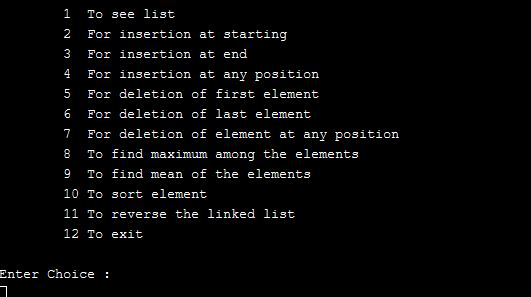Insertion at the starting: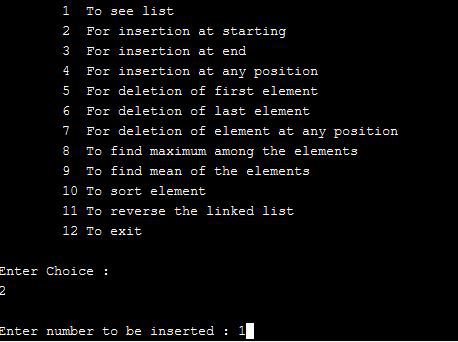Insertion at the end: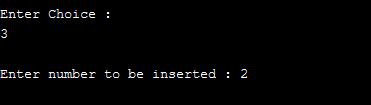Insertion at specific position: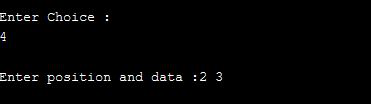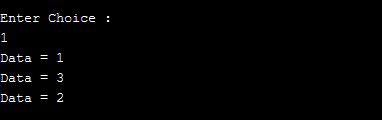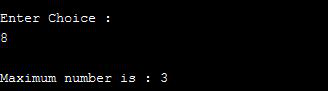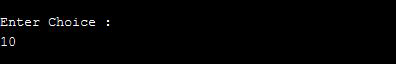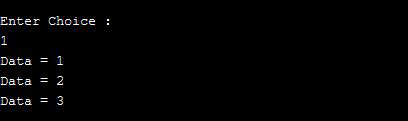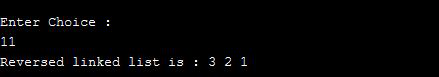Delete the first and last element with choice 5 and 6: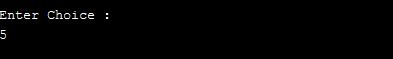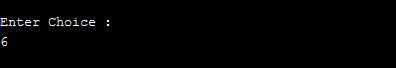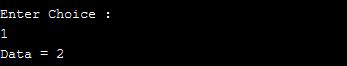My Personal Notes arrow_drop_up